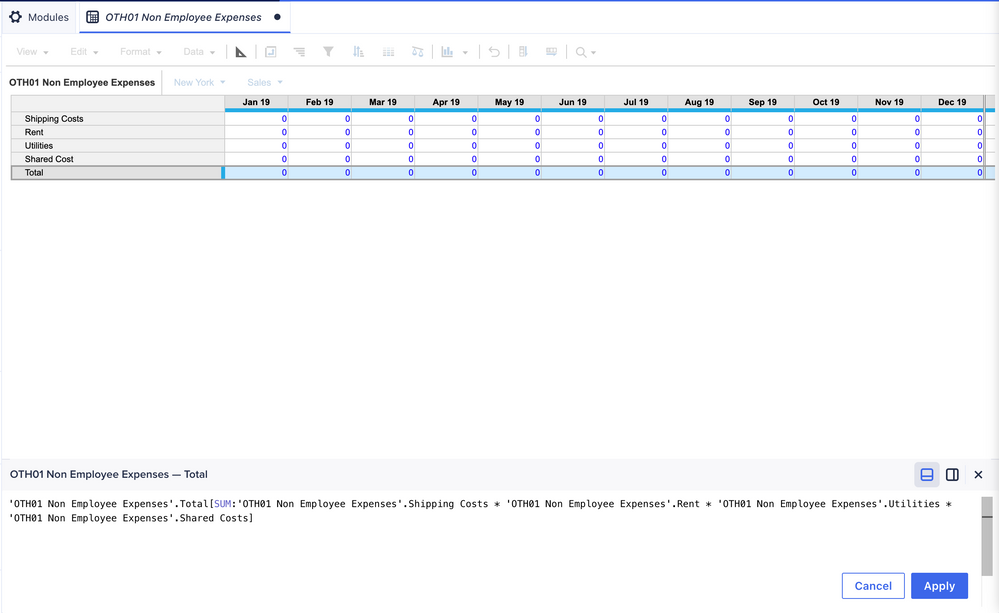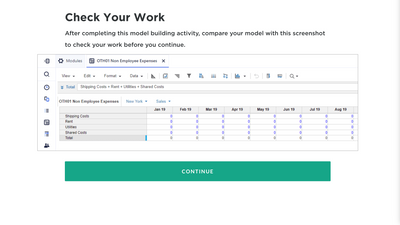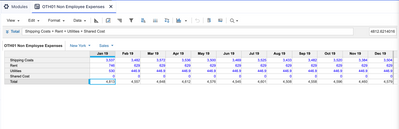# 10.2.1 Add non employee expenses moduleHi Community ! 😀

I'm learning the training and I completed everything but the formula is what holding me back for the past 30 minutes..

So below is what I have :& from the training  I am suppose to have ...•Hi

Try this formula. Its simply adding all costs

Shipping cost + Rent + Utilities + Shared Cost
As these are from the same module, no need to use modelename.lineitemname
•As I can see, you are putting the formula for Total line item. And Total formula will be addition of Shipping cost+rent+utilities+shared cost

So, it's simple addition formula. why  you are putting sum and all.

Shipping Costs + Rent + Utilities + Shared Costs

Hope it helps for you.

Thanks,

•Im sorry i still dont understand what you are saying. so the following formula you just put i insert

Shipping Costs + Rent + Utilities + Shared Costs

where does this formula go ?

•Look, You are putting the formula in TOTAL line item. So addition of all will be the total. So the formula will be just:

Shipping Costs + Rent + Utilities + Shared Costs

That's it.

Hope it helps,

Thanks

•So the line item "total" is addition of the the four above line items. So what @jagadishdash and @Sowjanyaivl have said absolutely right. It means you simply have to add the expenses to get "total" expense.

For your question: "where does this formula go ?" you will be referring the line item "total" in some other module for some other calculation. You should be able to figure it out during the course of the training.

•Hi Trisha,

Delete the formula which you have added earlier for linetem Total and add the new formula, then Apply

•I tried putting the formula in but it only accepts

Shipping Costs + Rent + Utilities ... not shared cost•@trishahazzardf  the new formula you added  is correct. And for shared cost you can see the import value is 0 so that's why it's adding the all 4 line items. Because of it's zero it's coming like this.

Check you import is correct or not.  The formula is fine.

Thanks,